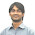Welcome! Dear Traders,you are reading my forex trading experiences. Forex trading is a very profitable and very risky business opportunity. If you are a beginner, calm down,have a cup of coffee, and convince yourself that you need to study hard to win in forex trading. Obviously, the task is not easy as the statistics claim that only 5% traders win in forex trading. If you are determined, serious,and hard working, you can surely be included in the group of winners.

### HOW TO TRADE BULLISH 5-0 HARMONIC CHART PATTERN

Bullish 5-0 harmonic chart pattern suggests a long entry upon completion of the pattern or confirming the D point of the pattern. This post explains the way to find or draw a Bullish 5-0 harmonic char pattern and the way to trade a Bullish 5-0 harmonic chart pattern.

How to draw or find a Bullish 5-0 harmonic chart pattern on price chart?
To draw or find a Bullish 5-0 harmonic chart pattern on price chart, first of all, we need to find the X and A points of the pattern. The X point is found at the bottom of a strong bearish trend, and the A point is found at the top of a pullback. Now we need to draw a fibonacci retracement tool from X to A to find the B point of the pattern. The B point should be within the 113%-161.8% fibonacci retracement of XA.

To find the B point without fibonacci retracement tool, we will use the formulas-

(IA) AB= (price at A point-price at X point) X 1.13
(IB) Price level at 113% of XA= (price at point A-result found from formula (IA))
(IIA) AB= (price at A point-price at X point) X 1.618
(IIB) Price level at 161.8% of XA= (price at point A-result found from formula (IIA))

In this case we can see, B point accurately satisfied the AB/XA= 1.13-1.618 condition of the pattern. See the illustration below.

In next step, we will draw a fibonacci retracement tool from A to B to find the C point. The C point should be within the 161.8%-224% fibonacci retracement of AB.

To find the C point without fibonacci retracement tool, we will use the formulas-

(IA) BC= (price at A point-price at B point) X 1.618
(IB) Price level at 161.8% of AB= (price at point B+result found from formula (IA))
(IIA) BC= (price at A point-price at B point) X 2.24
(IIB) Price level at 224% of AB= (price at point B+result found from formula (IIA))

The C point of this pattern should lie within the price levels found from formula (IB) and (IIB). This satisfies the BC/AB=1.618-2.24 condition of the pattern. Look at the following illustration.

In the final step, we will find the entry point, D point, of the bullish 5-0 harmonic chart pattern. To find the D point of the pattern, we will draw a fibonacci retracement tool from B to C. The D point should be at the 50% fibonacci retracement of BC.

To find the D point without fibonacci retracement tool, we will use the following formulas-

(IA) CD= (price at C point-price at B point) X 0.50
(IB) Price level at 50% of BC= (price at point C-result found from formula (IA))

The D point of this pattern should lie on the price level found from formula (IB). This satisfies the CD/BC=0.50 condition of the pattern. Look at the following illustration

How to trade the bullish 5-0 harmonic chart pattern?
A long entry is suggested when the D point of the pattern is confirmed. The stop loss for the order should be placed at the lower support level. The profit target for the order should be placed within the 50-88.6% fibonacci retracement of CD.

The C point can also be traded, if it is the D point of a bearish shark harmonic chart pattern. The B point can be traded, if the entries are confirmed by the other technical analysis tool(s).

Thank You and Good Luck.

1.2.